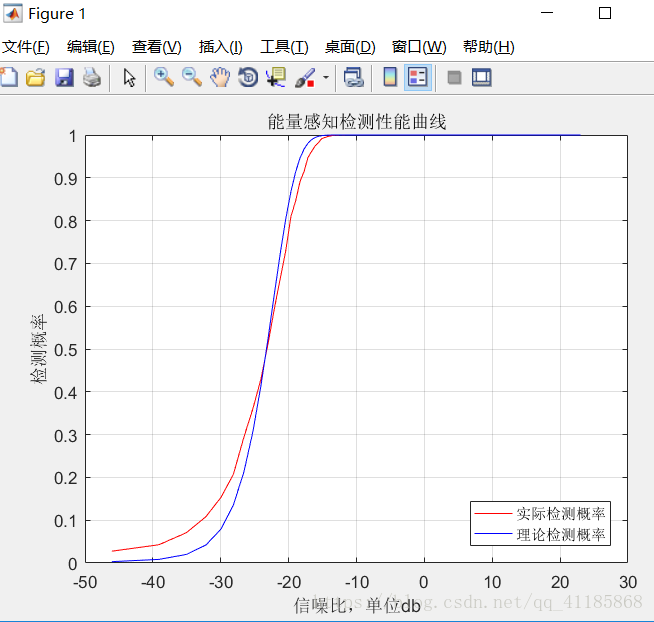# Matlab：绘制简单能量的接收机工作特性曲线(Energy_detection_simulation_ok)

+关注继续查看

## 输出结果## 实现代码

% This code is to plot receiver operating characteristic curve for simple energy

%绘制简单能量的接收机工作特性曲线

% detection, when the primary signal is real Gaussian signal and noise is

% addive white real Gaussian. Here, the threshold is available

% analytically.

% Code written by: Sanket Kalamkar, Indian Institute of Technology Kanpur,

% India.

%% 以下代码绘制在虚警概率一定时，检测概率和信噪比之间的关系曲线称为检测器的检测性能曲线

clc

close all

clear all

L = 1000;                                             % The number of samples

snr = 0.01:0.01:10;

Pf = 10e-4;                                           % Pf = Probability of False Alarm 虚警概率确定

%% Simulation to plot SNR vs.Probability of Detection (Pd)

for m = 1:length(snr)

i = 0;

thresh = (qfuncinv(Pf)./sqrt(L))+ 1;              % Theoretical value of Threshold, refer, Sensing-Throughput Tradeoff for Cognitive Radio Networks, Y. C. Liang

for kk = 1:5000                                   % Number of Monte Carlo Simulations(https://cn.mathworks.com/discovery/monte-carlo-simulation.html)

n = randn(1,L);                               % AWGN noise with mean 0 and variance 1

s = sqrt(snr(m)).*randn(1,L);                 % Real valued Gaussina Primary User Signal

y = s + n;                                    % Received signal at SU(认知用户接收到的信号)

energy = abs(y).^2;                           % Energy of received signal over N samples

energy_fin =(1/L).*sum(energy);               % Test Statistic for the energy detection

if(energy_fin >= thresh)                      % Check whether the received energy is greater than threshold, if so, increment Pd (Probability of detection) counter by 1

i = i+1;

end

end

Pd(m) = i/kk;

end

plot(10*log(snr), Pd,  'r')

xlabel('信噪比，单位db');

ylabel('检测概率');

title('能量感知检测性能曲线');

grid on

hold on

%% Theroretical expression of Probability of Detection; refer above reference.

thresh = (qfuncinv(Pf)./sqrt(L))+ 1;

%Pd_the = qfunc(((thresh - (snr + 1)).*sqrt(L))./(sqrt(2).*(snr + 1))); % 原来代码中的表达与论文中不一致

for k = 1:length(snr)

Pd_the(k) = qfunc(((thresh - (snr(k) + 1)).*sqrt(L))./(sqrt(2).*snr(k) + 1)); % 与论文中的方程式保持一致

end

plot(10*log(snr), Pd_the, 'b')

legend('实际检测概率', '理论检测概率', 'Location', 'SouthEast');

hold on

grid on167 0【对讲机的那点事】当对讲机不工作时如何处理？

942 03956 0SpringBoot中接收json数据及转换日期类型
2435 0【对讲机的那点事】450MHz模拟无线列调的工作原理（连载二）

1051 014093 0CSDN社区分享面试经历活动作品11——我的第一份实习工作

925 05676 0【对讲机的那点事】450MHz模拟无线列调的工作原理（连载四）

877 01803 0

1701

0

《SaaS模式云原生数据仓库应用场景实践》

《看见新力量：二》电子书